# Haste makes waste

• 权重w是控制输入信号的重要性的参数
• 阈值`θ`是调整神经元被激活的容易程度的参数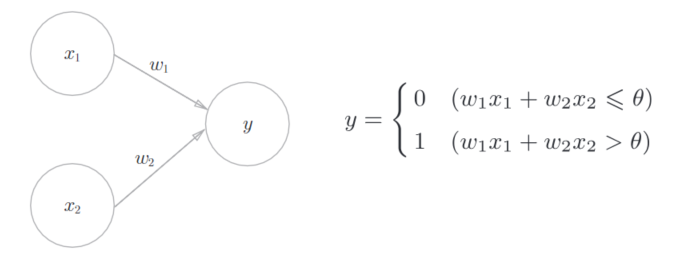# 1. 简单逻辑电路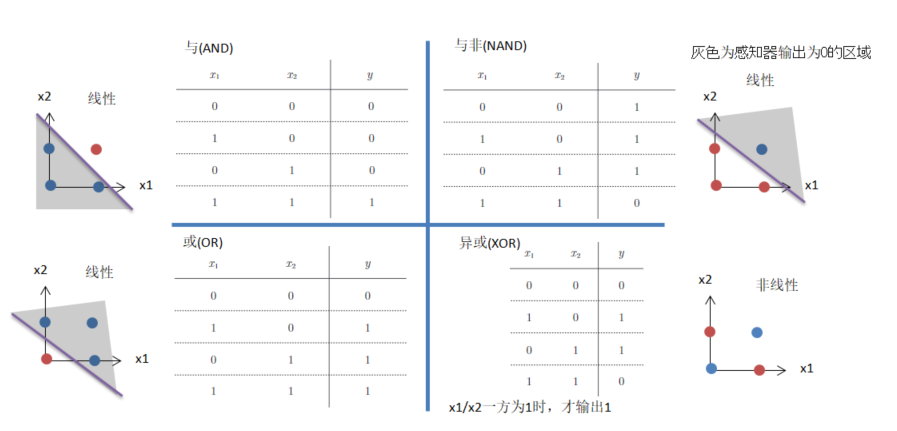# 2. 感知机的实现

``````import numpy as np
# 一般的实现
def AND1(x1,x2):
w1,w2,theta = 0.5,0.5,0.7
tmp = x1*w2 + x2*w2
if tmp <= theta:
return 0
elif tmp > theta:
return 1

# numpy矩阵实现
def AND2(x1,x2):
x = np.array([x1,x2])
w = np.array([0.5,0.5])
b = -0.7
tmp = np.sum(x*w) + b
if tmp<=0:
return 0
else:
return 1
``````

``````def NAND(x1,x2):
x = np.array([x1,x2])
w = np.array([-0.5,-0.5])
b = 0.7
tmp = np.sum(x*w) + b
if tmp<=0:
return 0
else:
return 1

def OR(x1,x2):
x = np.array([x1,x2])
w = np.array([0.5,0.5])
b = -0.2
tmp = np.sum(x*w) + b
if tmp<=0:
return 0
else:
return 1
``````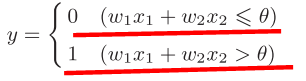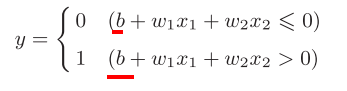1. 权重w1和w2是控制输入信号的重要性的参数，每个输入信号对应不同权重。
2. 偏置b是调整神经元被激活的容易程度的参数，一个神经元一个值。比如，如果b为-0.1，则只要输入信号的加权总和超过0.1，则神经元被激活。

# 3. 感知机的局限性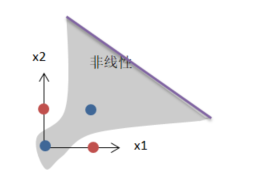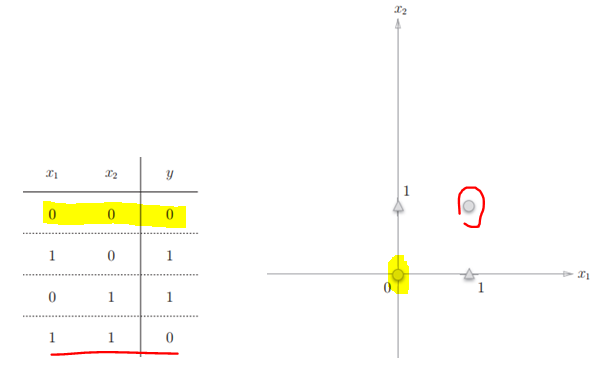# 4. 多层感知机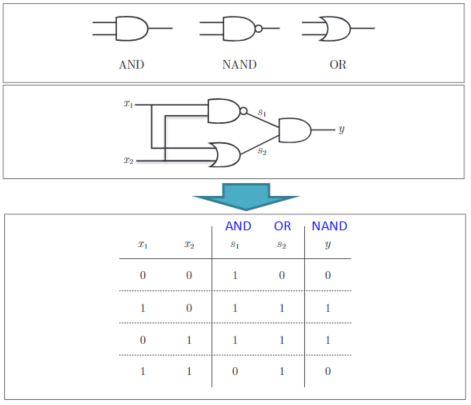``````def XOR(x1,x2):
s1 = NAND(x1,x2)
s2 = OR(x1,x2)
y = AND2(s1,s2)
return y

print(XOR(0,0))
print(XOR(1,0))
print(XOR(0,1))
print(XOR(1,1))

``````

``````0
1
1
0
``````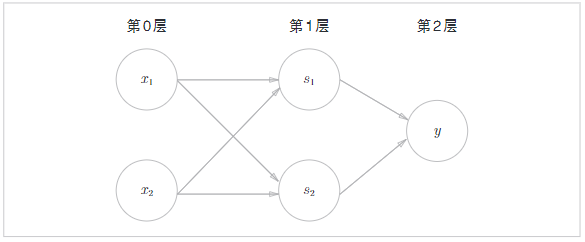1. 第0层的两个神经元接收输入信号，并将信号发送到第1层神经元
2. 第1层的神经元将信号发送至第2层神经元，第2层的神经元输出y

# 5. 小结

• 感知机是具有输入和输出的算法，给定一个输入后，将输出一个既定的值。
• 感知机将权重和阈值设定为参数。
• 使用感知机可以表示与/或等逻辑电路。
• 异或无法通过单层感知机来表示，可以通过二层感知机表示异或门。
• 单层感知器只能表示非线性空间，而多层感知机可以表示非线性空间。